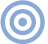Features

Bits is Bits? Page 2

We now proceed to model a bandwidth-limited link by filtering the subframe signal with a first-order (RC) low-pass filter, and determine what degree of filtering will result in bit errors. Using the first-order filter model is a gross simplification of the time-domain behavior of a real link—accurate analysis requires the use of transmission-line theory at the high frequencies involved—but it's a good starting point for investigation.

Consider the top section of fig.5, which shows a simulation of the subframe signal carrying an audio word value of 255 and filtered using a time constant of 100ns, corresponding to a -3dB frequency of 1.6MHz. (For all of the simulations and measurements presented in this paper the audio sampling rate is 44.1kHz.) Although the filtered subframe now has edges with finite rise and fall times, no transitions are missed; hence, no amplitude errors will occur as long as the receiver can latch the data correctly following transitions.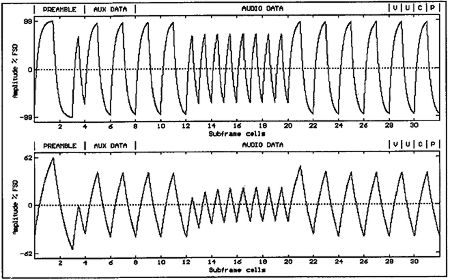Fig.5 Subframe signal filtered with time constant of 100ns (top) and 400ns (bottom).

The lower section of fig.5 indicates the same subframe, but filtered more severely with a 400ns time constant, corresponding to a -3dB frequency of 400kHz. In this simulation, the transition at the edge of cell 3 is missed; this will definitely result in a bit error in the decoded subframe. This example also indicates that receiver bit errors are most likely to occur during preambles where the largest variation in transition times occur (one half-cell width to three half-cell widths). This is interesting: many practical ADIC ICs indicate full lock to the received signal when the preambles are correctly detected; hence, if lock is achieved, bit errors are not likely.

However, most practical interface decoders will exhibit an upper time-constant lock limit considerably less than 400ns, due to the finite "time aperture" about the average zero-crossing point, during which a transition is allowed without latched data errors. In "Jitter in the Digital Audio Interface" (below), we derive an expression for the peak-to-peak variation in zero-crossing times tx in terms of the range of interface signal pulse widths. If we let the large pulse width be 3tc/2 and the smaller be tc/2 (where tc is the cell width in the biphase-mark coding) then (cf Equation.11):

Equation 1: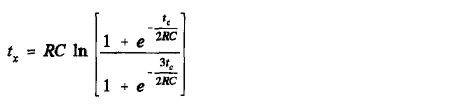Using the experimental ADIC circuit shown in fig.4, full signal lock was achieved for interface time constants up to RC = 170ns, indicating that for this particular example the maximum zero-crossing-time aperture tx is approximately 45ns. In practice, time constants greater than 100ns are excessive for digital links, which should be designed with bandwidths well above 6MHz. We have measured 2-meter interface links with a characteristic impedance of 75 ohms, correctly terminated both at the transmitter and receiver, with 10-90% rise and fall times of <10ns. This performance level corresponds to a 35MHz bandwidth.

In this section we have shown that bit errors in the received subframe occur when transitions in the interface signal are incorrectly latched. This will not occur in most interface receivers for interface time constants of <100ns. Bit errors that do occur will most likely be in the preamble, and will usually result in the receiver failing to lock onto the incoming signal.

Audio bit errors due to band limitation alone are extremely unlikely. Of course, this simple analysis does not consider the effects of noise on the bandwidth-limited link. As the bandwidth of the link decreases, the eye-pattern representation of the received signal suffers from a decreasing opening. This results in more time spent in the threshold region, and the probability of noise-induced errors increases. Nevertheless, Cabot (footnote 5) presents an interface example in which bit errors are negligible for noise levels up to 20dB below the interface signal level with RC filtering up to 160ns, and claims to have achieved zero error-rate transmissions over an unmatched digital audio link of 100m length.

Jitter in the digital audio interface
The second interface-error mechanism to consider is that of the recovered clock frequency's modulation. If the clock signal fed to an ideal DAC varies in frequency, the reconstructed analog output from the DAC will include error artifacts, even if the sample values fed to the DAC are correct. The easiest way to analyze such an effect is by examining the jitter on the recovered clock. (In this article, we define jitter as the instantaneous timing deviation of clock transitions from their correct positions.)

Interface Bandwidth Limitation: Consider the subframe carrying an audio word value of 255 in fig.6. In the upper diagram, the unfiltered subframe represents the signal transmitted; in the lower diagram, the received signal at the interface decoder has been filtered with an RC time constant of 200ns. If we define zero-crossing time tx as the time taken for the received interface signal to cross the 0V detection axis after a transition has occurred at the transmitter, then tx depends upon the voltage at the receiver at transmitter transition time, and inter-symbol interference occurs; ie, zero-crossing time depends on the values of previous pulse widths.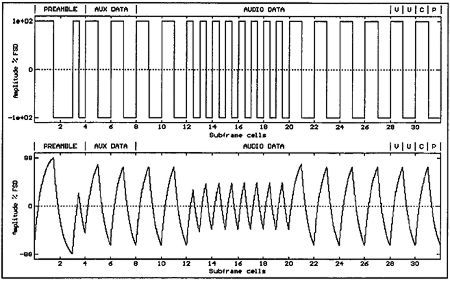Fig.6 Subframe signal unfiltered (top) and filtered with time constant of 200ns (bottom).

This phenomenon is shown more clearly in fig.7, which has an expanded time scale; the zero-crossing time at the end of cell 4 is smaller than that at the end of cell 6 (where the voltage at the receiver has had time to fall to a lower value). When both transmitted and received signals are known, we can compute the change in zero-crossing times at each subframe cell boundary by searching for the change in polarity of the filtered signal.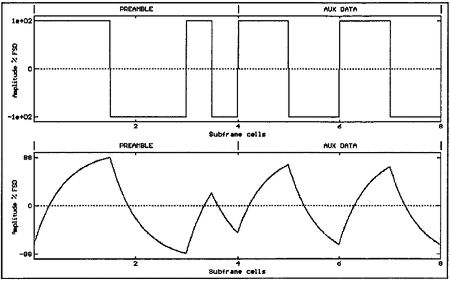Fig.7 Subframe signal unfiltered (top) and filtered with time constant of 200ns (bottom) (expanded time scale).

A simple computer program was written to perform this task, using the filtered data shown in the bottom half of fig.6. The calculated results (fig.8) indicate that in this example, the zero-crossing-time variation across the filtered subframe is about 50ns; when a series of ones are transmitted, the peak voltage received at the end of the interface falls, resulting in a reduction in zero-crossing time. The variation in zero-crossing time results in a modulation of edge timing in the clock recovered from the interface signal, and this edge modulation is clearly dependent upon the number of ones and zeros transmitted in each subframe: instantaneous recovered clock jitter is dependent upon the audio-word value transmitted over the interface.Fig.8 Variation in cell-edge zero-crossing times across the subframe signal shown in the bottom of fig.6.

We will now develop an expression for the detected zero-crossing time at a given transition with a known history of previous transition times. Consider fig.9, which shows the exponential rise of a filtered transition with time-constant RC. The transmitted signal has a peak "driving" voltage Vd, while the received voltage has an initial value V0 at the time of the transition. If we denote the transition time as 0s, then the behavior of the received signal following the transition can be described by a simple exponential time equation: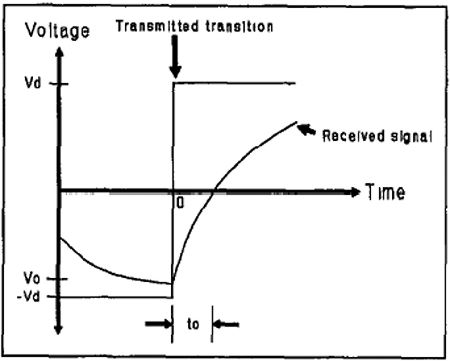Fig.9 Exponential rise of filtered interface signal following transmission.

Equation 2: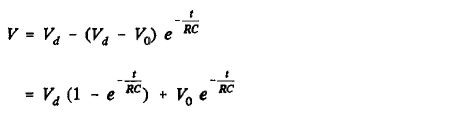Setting V to zero and rearranging gives the zero-crossing time:

Equation 3: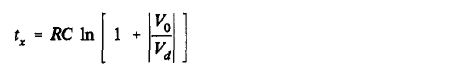The zero-crossing time evidently has a dependency upon the initial voltage V0 at transition time, and this in turn will depend upon previous pulse widths. If the previous transition in the interface signal occurred at -t1 seconds, then V0 can be written in terms of t1 and V1 (the voltage at the previous transition):

Equation 4: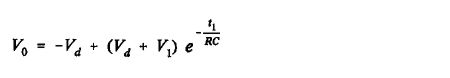Substituting into Equation 2:

Equation 5: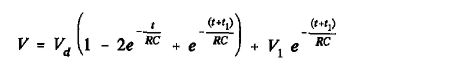This process can be continued with the next transition time at -(t1+t2) seconds, the next at -(t1+t2+t3) etc., to give:

Equation 6: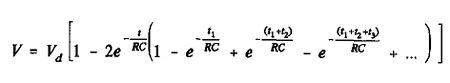Hence, we can write the zero-crossing time tx for the transition at 0 s in terms of the previous pulse widths t1, t2, t3...:

Equation 7: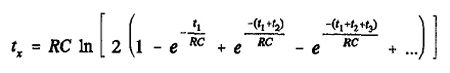Using Equation 7, we can now compute the zero-crossing time at each transition in a filtered interface signal containing several subframes. This yields a signal with a sampling rate equal to the maximum rate of interface transitions; ie, 5.6MHz. In our simulations, we want to map the zero-crossing times of the filtered interface signal to jitter on the recovered clock at the output of the ADIC. However, 5.6MHz is too high a sampling rate with which to efficiently compute the effect of such a jitter signal upon any reasonable length of audio data.

Footnote 5: R.C. Cabot, "Measuring AES/EBU Digital Audio Interfaces," presented at the 87th AES Convention, New York, October 1989, Preprint 2819.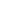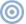ARTICLE CONTENTSX